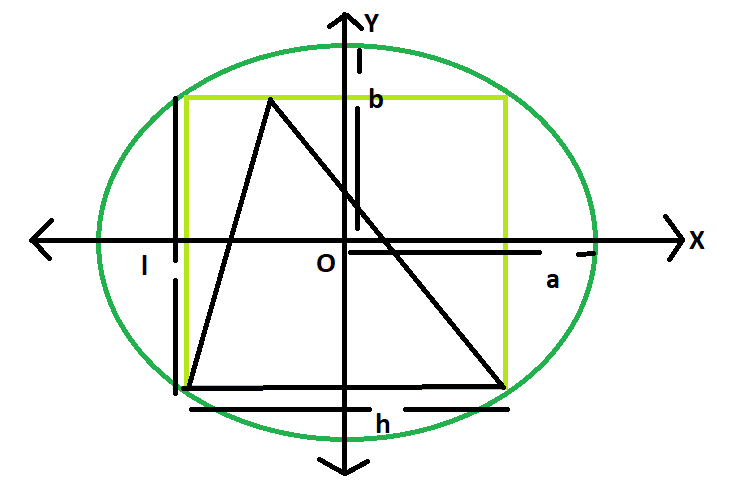# Area of a triangle inscribed in a rectangle which is inscribed in an ellipse

Given here is an ellipse with axes length 2a and 2b, which inscribes a rectangle of length l and breadth h, which in turn inscribes a triangle.The task is to find the area of this triangle.
Examples:

```Input: a = 4, b = 3
Output: 12

Input: a = 5, b = 2
Output: 10```Approach
We know the Area of the rectangle inscribed within the ellipse is, Ar = 2ab(Please refer here),
also the area of the triangle inscribed within the rectangle s, A = Ar/2 = ab(Please refer here)
Below is the implementation of the above approach:

## C++

 `// C++ Program to find the area of the triangle` `// inscribed within the rectangle which in turn` `// is inscribed in an ellipse` `#include ` `using` `namespace` `std;`   `// Function to find the area of the triangle` `float` `area(``float` `a, ``float` `b)` `{`   `    ``// length of a and b cannot be negative` `    ``if` `(a < 0 || b < 0)` `        ``return` `-1;`   `    ``// area of the triangle` `    ``float` `A = a * b;` `    ``return` `A;` `}`   `// Driver code` `int` `main()` `{` `    ``float` `a = 5, b = 2;` `    ``cout << area(a, b) << endl;` `    ``return` `0;` `}`

## Java

 `   ``// Java Program to find the area of the triangle` `// inscribed within the rectangle which in turn` `// is inscribed in an ellipse`   `import` `java.io.*;`   `class` `GFG {`   `// Function to find the area of the triangle` `static` `float` `area(``float` `a, ``float` `b)` `{`   `    ``// length of a and b cannot be negative` `    ``if` `(a < ``0` `|| b < ``0``)` `        ``return` `-``1``;`   `    ``// area of the triangle` `    ``float` `A = a * b;` `    ``return` `A;` `}`   `// Driver code`     `    ``public` `static` `void` `main (String[] args) {` `    ``float` `a = ``5``, b = ``2``;` `    ``System.out.println(area(a, b));` `    ``}` `}` `//This code is contributed by anuj_67..`

## Python3

 `# Python 3 Program to find the ` `# area of the triangle inscribed ` `# within the rectangle which in ` `# turn is inscribed in an ellipse`   `# Function to find the area` `# of the triangle` `def` `area(a, b):` `    `  `    ``# length of a and b cannot ` `    ``# be negative` `    ``if` `(a < ``0` `or` `b < ``0``):` `        ``return` `-``1`   `    ``# area of the triangle` `    ``A ``=` `a ``*` `b` `    ``return` `A`   `# Driver code` `if` `__name__ ``=``=` `'__main__'``:` `    ``a ``=` `5` `    ``b ``=` `2` `    ``print``(area(a, b))` `    `  `# This code is contributed ` `# by Surendra_Gangwar`

## C#

 `// C# Program to find the area of ` `// the triangle inscribed within ` `// the rectangle which in turn` `// is inscribed in an ellipse` `using` `System;`   `class` `GFG` `{` `    `  `// Function to find the ` `// area of the triangle` `static` `float` `area(``float` `a, ``float` `b)` `{`   `    ``// length of a and b ` `    ``// cannot be negative` `    ``if` `(a < 0 || b < 0)` `        ``return` `-1;`   `    ``// area of the triangle` `    ``float` `A = a * b;` `    ``return` `A;` `}`   `// Driver code` `static` `public` `void` `Main ()` `{` `    ``float` `a = 5, b = 2;` `    ``Console.WriteLine(area(a, b));` `}` `}`   `// This code is contributed by ajit`

## PHP

 ``

## Javascript

 ``

Output:

`10`

Time complexity: O(1)

Auxiliary Space: O(1)

Feeling lost in the world of random DSA topics, wasting time without progress? It's time for a change! Join our DSA course, where we'll guide you on an exciting journey to master DSA efficiently and on schedule.
Ready to dive in? Explore our Free Demo Content and join our DSA course, trusted by over 100,000 geeks!

Previous
Next